﻿ Analyzing Numerical Input
Liquid Studio Documentation
Data Mapping / Analyzing Numerical Input
In This Topic
Analyzing Numerical Input
In This Topic

It goes without saying that the data mapping process often involves manipulations of data values, rather than straight translations to new Schema structures. The mapping functions are designed to facilitate this process. Among the functions, particularly in the Maths section, there are a number of options you can use to analyse numerical input. Using these, your output data can include processed information based on the content of the input data. In this section, we will demonstrate a typical case in which the output data comprises an analysis of some aspect of the input source.

Create a new Data Mapper file, dragging your XML data source and targets into the editor area. For this example we are using payroll_source.xsd, an XML Schema Definition inferred from the following source: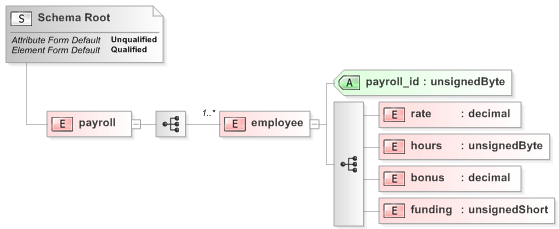Source XML
Copy Code
```<payroll>
<employee payroll_id="126">
<rate>9.45</rate>
<hours>35</hours>
<bonus>70.00</bonus>
<funding>350</funding>
</employee>
<employee payroll_id="129">
<rate>10.50</rate>
<hours>30</hours>
<bonus>150.00</bonus>
<funding>500</funding>
</employee>
<employee payroll_id="130">
<rate>8.90</rate>
<hours>40</hours>
<bonus>100.00</bonus>
<funding>550</funding>
</employee>
<employee payroll_id="133">
<rate>11.20</rate>
<hours>40</hours>
<bonus>200.00</bonus>
<funding>600</funding>
</employee>
</payroll>
```

For our target schema we will be using payroll_target.xsd, inferred from the following XML: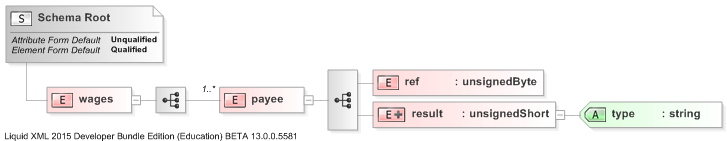Target XML
Copy Code
```<wages>
<payee>
<ref>122</ref>
<result type="remainder">30 full hours with a remainder of £45</result>
</payee>
<payee>
<ref>125</ref>
<result type="remainder">40 full hours with a remainder or £30</result>
</payee>
<payee>
<ref>126</ref>
<result type="remainder">35 full hours with a remainder of £25</result>
</payee>
</wages>
```

The XML models payroll data for temporary staff within an organisation. The output is designed to capture some value calculated from the source, using the "type" attribute to indicate what the calculation result represents. Let's assume that we want to calculate the number of full hours each employee is budgeted for, and the remainder after that. We will need to carry out division and subtraction calculations to work out the weekly funding budget, before applying the Modulus function in conjunction with Cast and String functions. Here is the Data Mapper with source and target imported:Drag the Maths Modulus function from the Component Palette into the mapping area: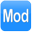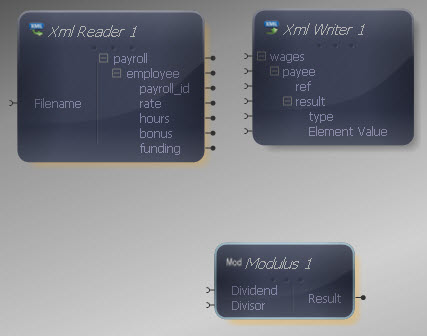We will use the Modulus component to calculate the remainder of the budgeted funding for each employee after full hours are deducted. First, we need to calculate the weekly funding budget to work from, which will be the full funding amount minus the bonus. Drag a Subtract function onto the Mapper, connecting its first input to the "funding" output in the XML Reader and its second input to the "bonus" output.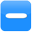Connect the output of the Subtract function to the Dividend input in the Modulus function. Next connect the "rate" output in the XML Reader to the "divisor" input in the Modulus function.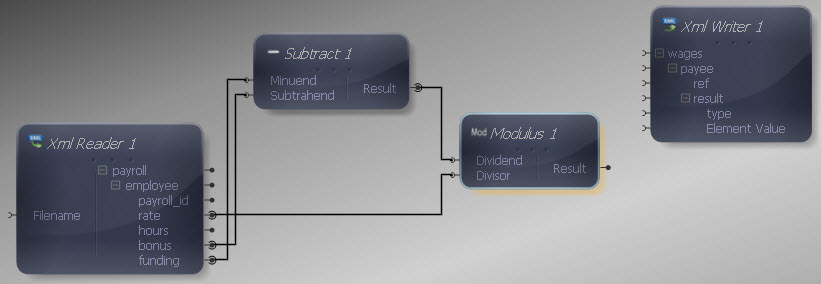Now we want to calculate the amount of hours paid for in addition to the remainder, so drag a Divide component onto the Mapper. Connect its first input to the output of the Subtract function and its second input to the "rate" output in the XML Reader, the same as what we did for the Modulus component itself.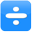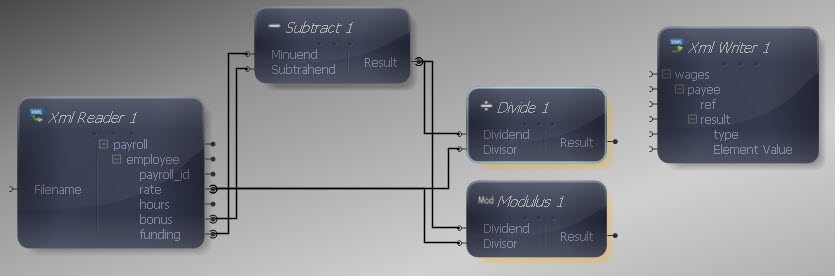Now we want to discard the fractional part of the division result, as we are only interested in full hours, with the Modulus result representing the rest of the calculation. Let's cast the division result to an integer for this reason. Drag a Cast function from the Type section onto the Mapper. Right-click it and choose Show Properties, selecting an integer type.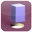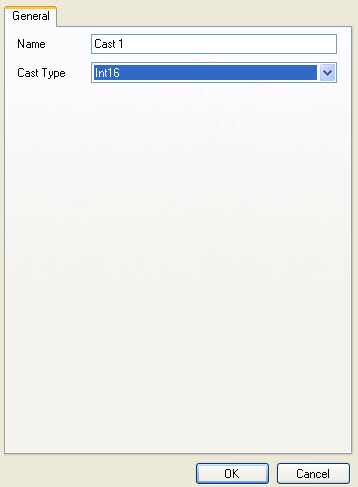Connect the Cast input to the output of the Divide function.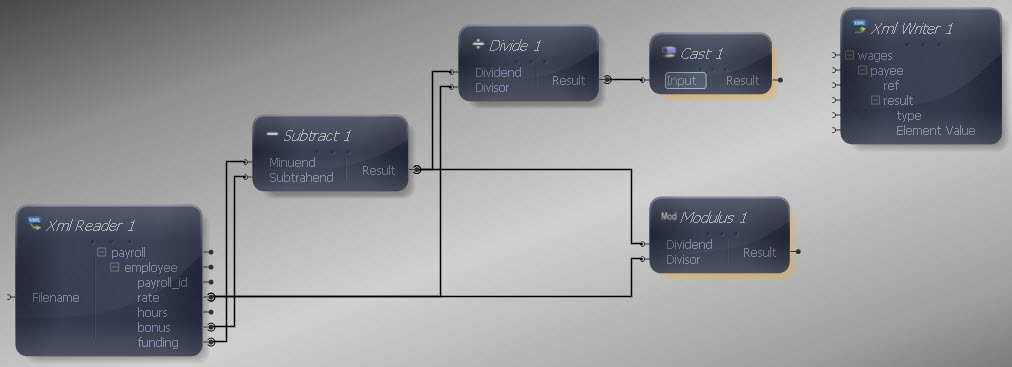Now let's concatenate the results of these calculations into a String. Drag a ConcatAll function onto the Mapper, connecting its first String input to the output of the Cast component.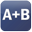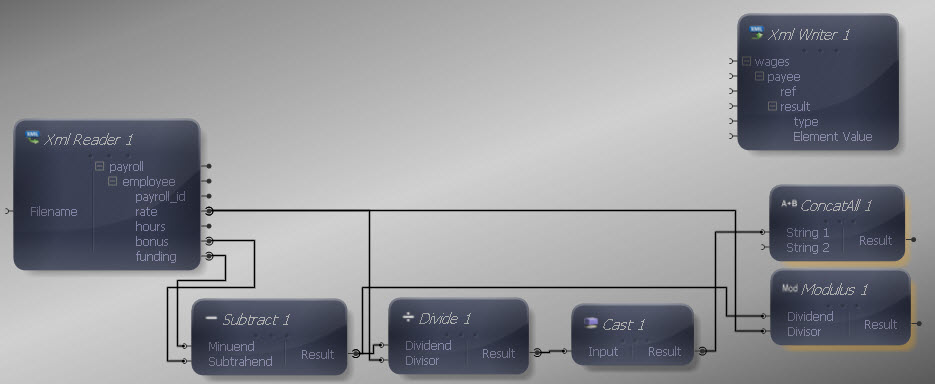Now for the second part of our String we want to define some text, so drag a Constant Value onto the Mapper from the Data Type section. Right-click it, choosing Show Properties. Select a String Data Type and enter the following text as the Value: " full hours with a remainder of £".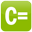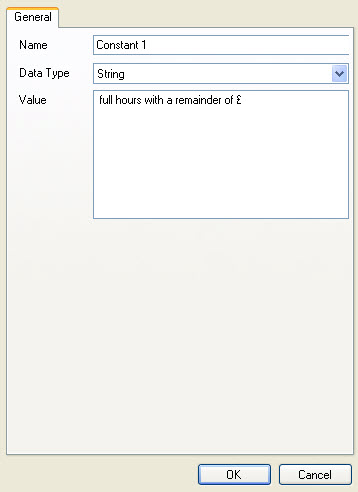Connect the Constant output to the second ConcatAll input.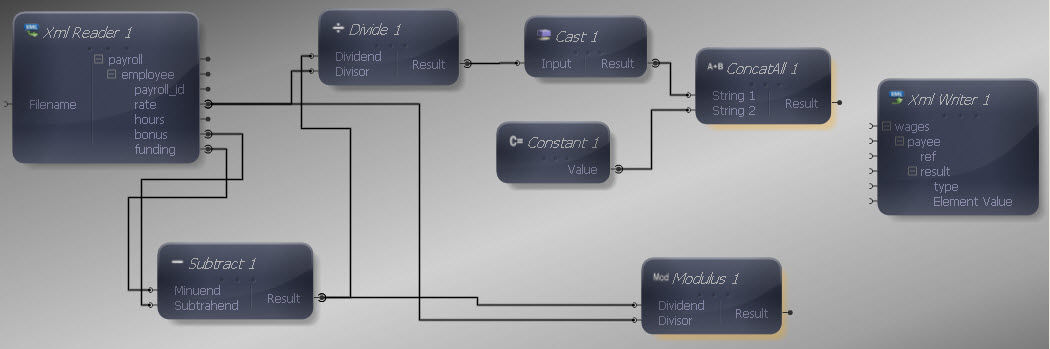Now we need to add another String to the ConcatAll function, so right-click its second input, choosing Add Below.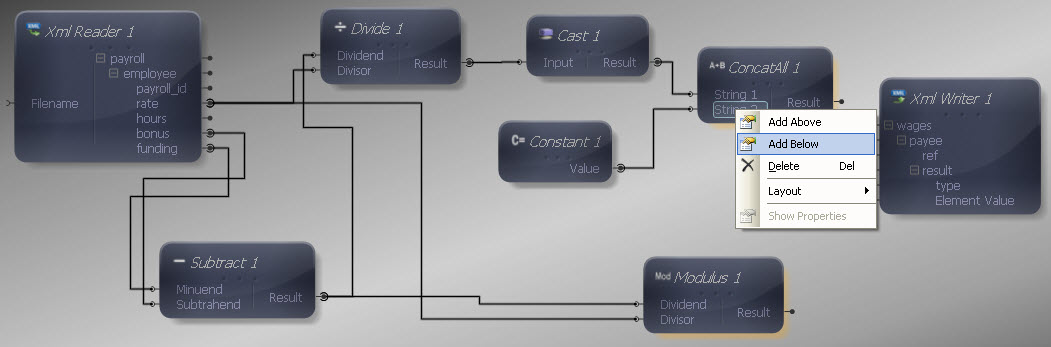Connect the output of the Modulus function to the third ConcatAll input.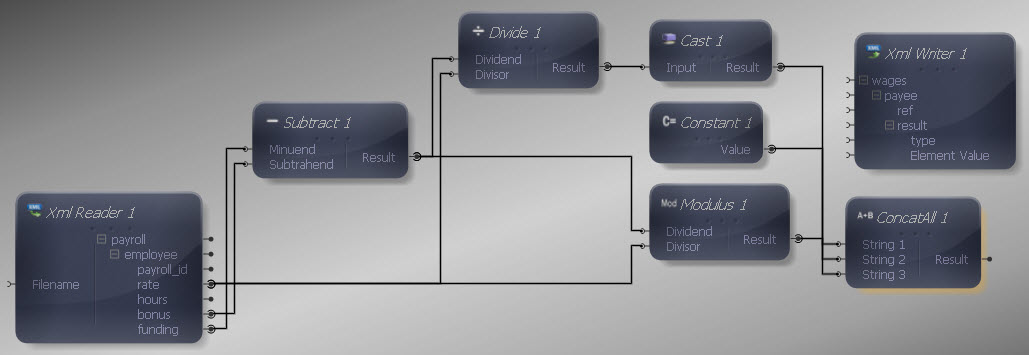Now connect the ConcatAll output to the "Element Value" input in the XML Writer, so that the concatenated String containing the calculation results is written into the "result" element.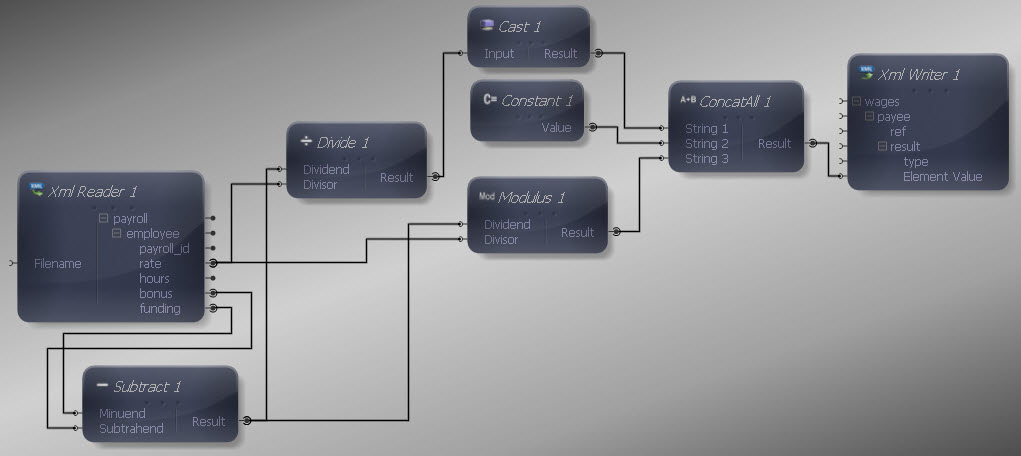Now we need to include the "type" attribute in the source, so add a Constant Value from the Data Type section. Right-click it and choose Show Properties. Choose a String Data Type and enter "remainder" as the value.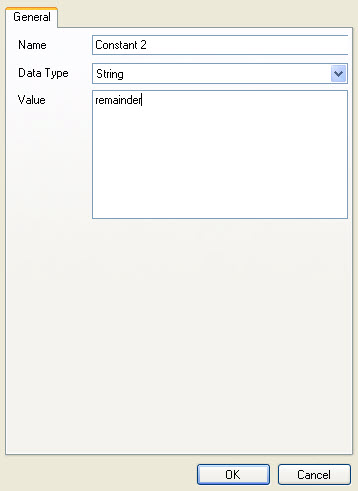Connect the Constant output to the "type" input in the XML Writer.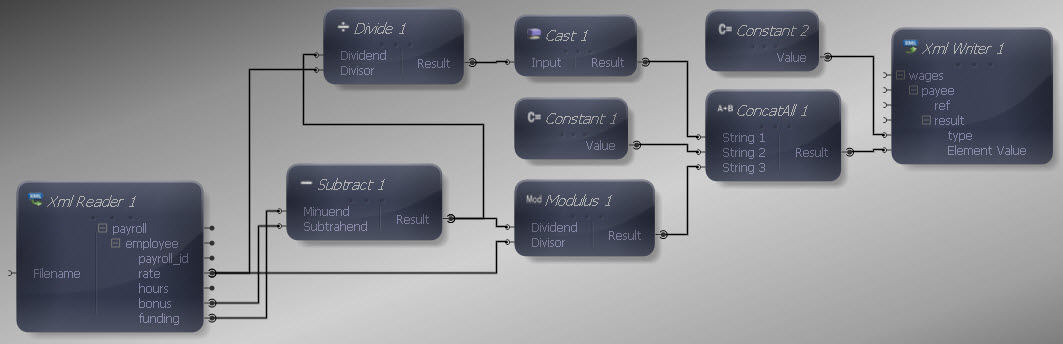Finally let's make the remaining input and output connections. We do not want to map all data items in the source, as we are only interested in certain values. Map "payroll" to "wages", "employee" to "payee" and "payroll_id" to "ref". We don't need to map anything to the "result" element as it will be written out each time the Mapper maps the addition result to it. Although we have used some of the remaining input values for calculations, we do not need to map them individually to the target, as we only want the calculation result.

We can now execute the transform by pressing Shift-F5 or the Execute button (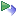). The transform is applied and the file we selected as output opens in the editor:

Output XML
Copy Code
```<wages>
<payee>
<ref>126</ref>
<result type="remainder">30 full hours with a remainder of £5.95</result>
</payee>
<payee>
<ref>129</ref>
<result type="remainder">33 full hours with a remainder of £3.50</result>
</payee>
<payee>
<ref>130</ref>
<result type="remainder">51 full hours with a remainder of £5.00</result>
</payee>
<payee>
<ref>133</ref>
<result type="remainder">36 full hours with a remainder of £8.00</result>
</payee>
</wages>
```

The output contains a String indicating the number of full hours and remaining budget for each employee. We have analysed the input data values during mapping, with the output containing a human-readable indicator of this processing. The transform functions therefore allow you to add a level of computation into your mapping activity, for example using output data for reporting purposes.